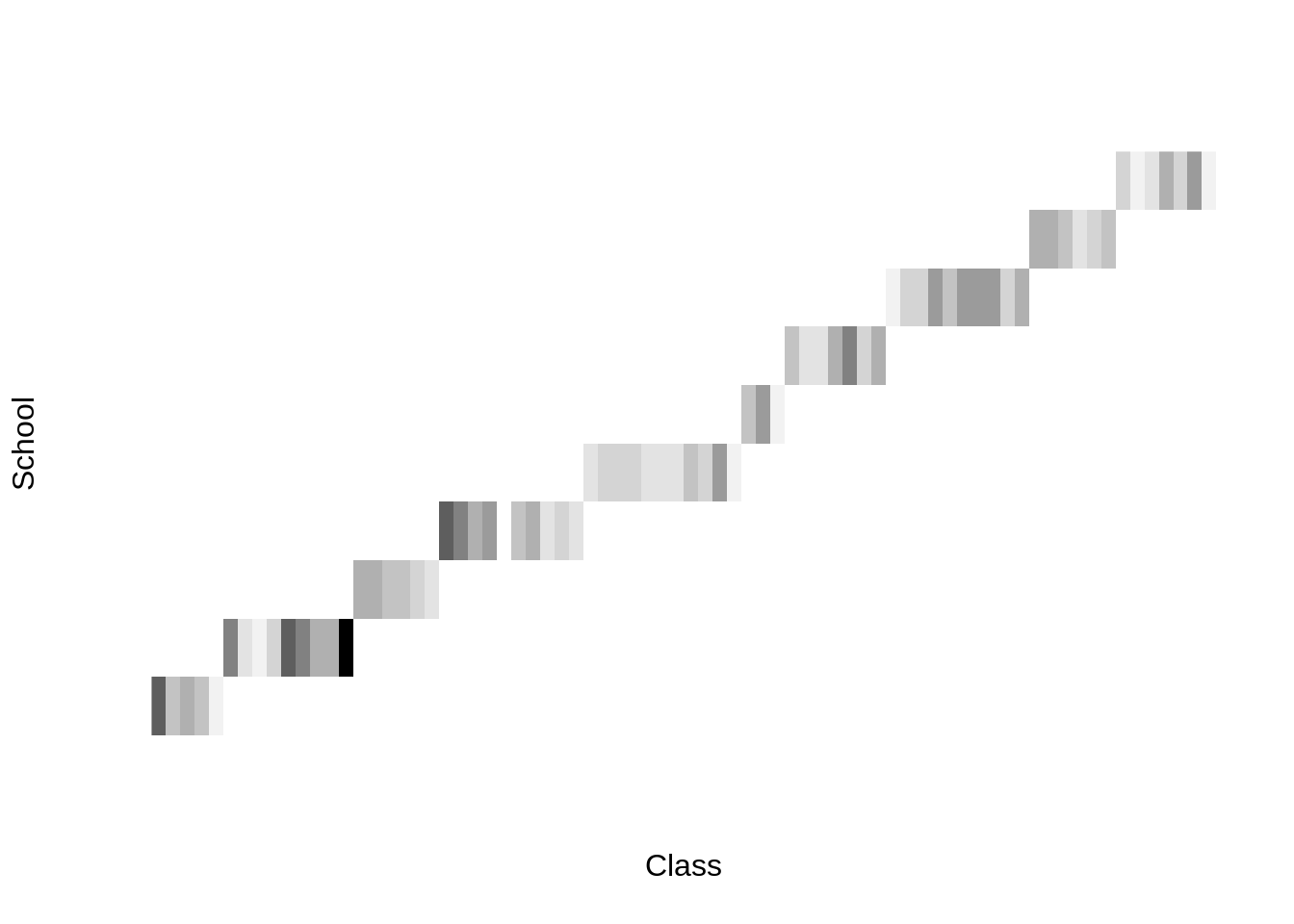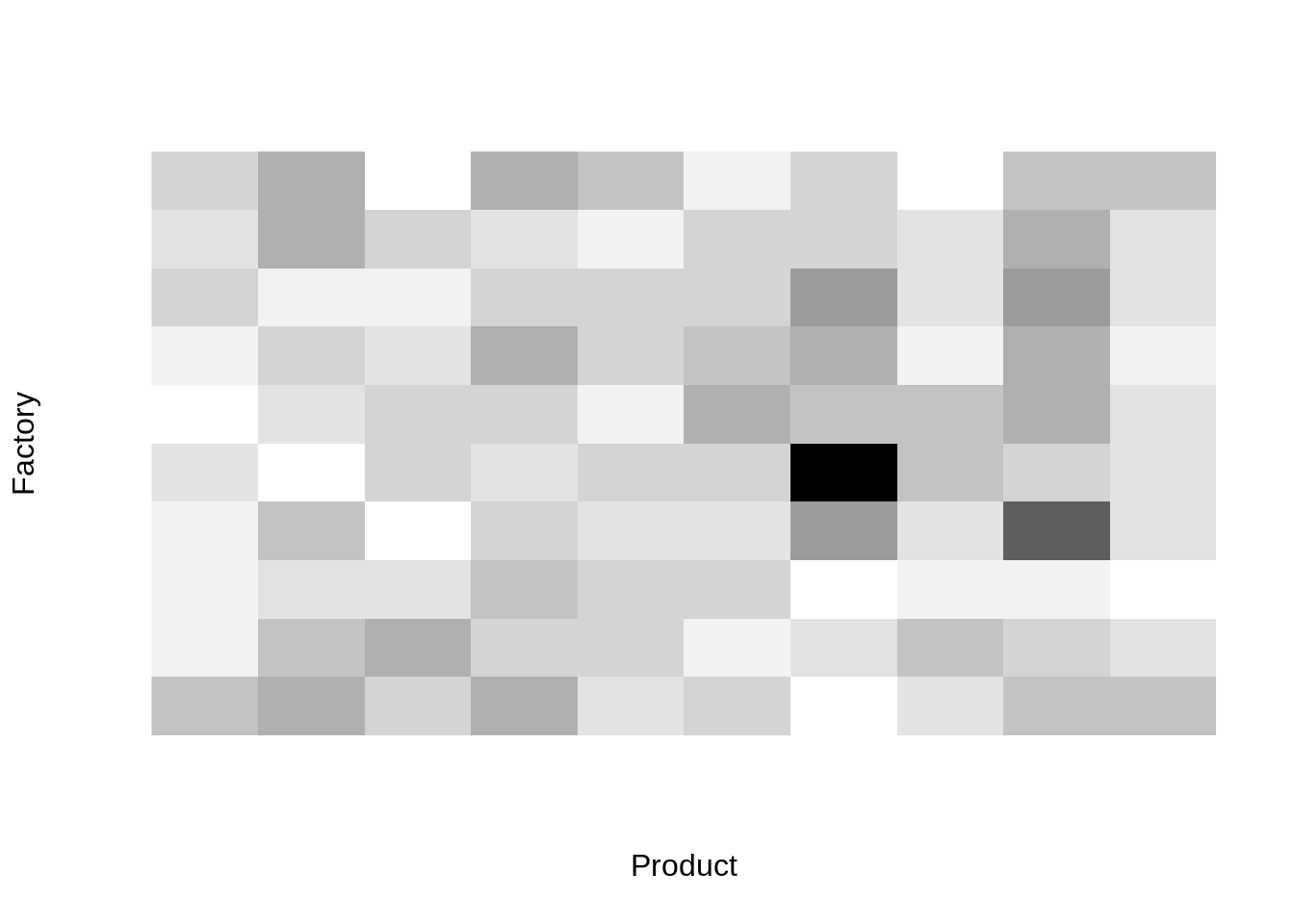# Nested and crossed random effects in "lme4"

People often get confused on how to code nested and crossed random effects in the lme4 package. I will try to make this more clear using some artificial data sets.

## Nested random effects

Nested random effects assume that there is some kind of hierarchy in the grouping of the observations. E.g. schools and classes. A class groups a number of students and a school groups a number of classes. There is a one-to-many relationship between the random effects. E.g. a school can contain multiple classes but a class can only be part of one school. Lets start by creating a simple example with fake data to explain the design. Figure 1 shows the contigency matrix for the dataset.

library(DT)
library(lme4)
library(tidyverse)
set.seed(123)
n_school <- 10
mean_n_class <- 7
mean_n_student <- 5

n_class <- rpois(n_school, mean_n_class)
schools <- map2_df(
seq_len(n_school),
n_class,
~data_frame(
school = .x,
class = seq_len(.y),
students = rpois(.y, mean_n_student)
)
) %>%
group_by(school, class) %>%
do(
student = data_frame(student = seq_len(.$students)) ) %>% unnest(student) %>% mutate( class2 = interaction(class, school, drop = TRUE), student2 = interaction(class2, student, drop = TRUE) ) schools contains 3 design variables: school, class and student. Each numbering restarts at 1 when the higher level number changes. Hence the id of class and student are not unique. Therefore I added two new variables class2 and student2 which are unique id’s for each class and student. The next step is adding the expected and observed values. with(schools, table(class2, school)) %>% image( col = grey.colors(10, start = 1, end = 0), axes = FALSE, xlab = "Class", ylab = "School" )Figure 1: Contingency matrix for the schoolsdata set school_sd <- 2 class_sd <- 2 noise_sd <- 1 intercept <- 50 school_effect <- rnorm(n_school, mean = 0, sd = school_sd) class_effect <- rnorm(length(levels(schools$class2)), mean = 0, sd = class_sd)
schools <- schools %>%
mutate(
mu = intercept + school_effect[school] + class_effect[class2],
y = mu + rnorm(n(), mean = 0, sd = noise_sd)
)

### Explicit nesting

The first option is to use explicit nesting. Here we add a random effect for each hierarchical level and use the : notation to add all higher levels. This can be expanded to more than two levels. E.g. (1|A) + (1|A:B) + (1|A:B:C) + (1|A:B:C:D). The nice thing about this notation is twofold: a) the nesting is explicit and clear for all readers; b) it is insensitive for the order: e.g. (1|A) + (1|A:B) is identical to (1|B:A) + (1|A).

lmer(y ~ (1|school) + (1|school:class), data = schools)
## Linear mixed model fit by REML ['lmerMod']
## Formula: y ~ (1 | school) + (1 | school:class)
##    Data: schools
## REML criterion at convergence: 1290.668
## Random effects:
##  Groups       Name        Std.Dev.
##  school:class (Intercept) 1.631
##  school       (Intercept) 1.806
##  Residual                 1.073
## Number of obs: 366, groups:  school:class, 74; school, 10
## Fixed Effects:
## (Intercept)
##       50.27
lmer(y ~ (1|class:school) + (1|school), data = schools)
## Linear mixed model fit by REML ['lmerMod']
## Formula: y ~ (1 | class:school) + (1 | school)
##    Data: schools
## REML criterion at convergence: 1290.668
## Random effects:
##  Groups       Name        Std.Dev.
##  class:school (Intercept) 1.631
##  school       (Intercept) 1.806
##  Residual                 1.073
## Number of obs: 366, groups:  class:school, 74; school, 10
## Fixed Effects:
## (Intercept)
##       50.27

### Shorthand nesting

(1|A) + (1|A:B) can be abbreviated into (1|A/B). However, I recommend against it because here the order is important as seen in the example below. (1|B/A) expands to (1|B) + (1|B:A), which is clearly a different model than (1|A) + (1|A:B). I’ve seen many people being confused about the order, therefore I recommend to be explicit instead of using shorthand.

lmer(y ~ (1|school/class), data = schools)
## Linear mixed model fit by REML ['lmerMod']
## Formula: y ~ (1 | school/class)
##    Data: schools
## REML criterion at convergence: 1290.668
## Random effects:
##  Groups       Name        Std.Dev.
##  class:school (Intercept) 1.631
##  school       (Intercept) 1.806
##  Residual                 1.073
## Number of obs: 366, groups:  class:school, 74; school, 10
## Fixed Effects:
## (Intercept)
##       50.27
lmer(y ~ (1|class/school), data = schools)
## Linear mixed model fit by REML ['lmerMod']
## Formula: y ~ (1 | class/school)
##    Data: schools
## REML criterion at convergence: 1320.039
## Random effects:
##  Groups       Name        Std.Dev.
##  school:class (Intercept) 2.330
##  class        (Intercept) 0.000
##  Residual                 1.073
## Number of obs: 366, groups:  school:class, 74; class, 11
## Fixed Effects:
## (Intercept)
##       50.42

### Implicit nesting

With implicit nesting, the nesting is ‘defined’ in the data. That is each level of a random effect has a one-to-many relation with the levels of the lower random effect. E.g. each class id is unique for a given class in a given school and cannot refer to a class in any other school. This is how we constructed the class2 variable in our data. With implicit nesting the code can be abbreviated to (1|A) + (1|B). Note that the (1|A) + (1|A:B) and (1|A/B) notations remain valid.

lmer(y ~ (1|school) + (1|class2), data = schools)
## Linear mixed model fit by REML ['lmerMod']
## Formula: y ~ (1 | school) + (1 | class2)
##    Data: schools
## REML criterion at convergence: 1290.668
## Random effects:
##  Groups   Name        Std.Dev.
##  class2   (Intercept) 1.631
##  school   (Intercept) 1.806
##  Residual             1.073
## Number of obs: 366, groups:  class2, 74; school, 10
## Fixed Effects:
## (Intercept)
##       50.27
lmer(y ~ (1|school) + (1|school:class2), data = schools)
## Linear mixed model fit by REML ['lmerMod']
## Formula: y ~ (1 | school) + (1 | school:class2)
##    Data: schools
## REML criterion at convergence: 1290.668
## Random effects:
##  Groups        Name        Std.Dev.
##  school:class2 (Intercept) 1.631
##  school        (Intercept) 1.806
##  Residual                  1.073
## Number of obs: 366, groups:  school:class2, 74; school, 10
## Fixed Effects:
## (Intercept)
##       50.27
lmer(y ~ (1|school/class2), data = schools)
## Linear mixed model fit by REML ['lmerMod']
## Formula: y ~ (1 | school/class2)
##    Data: schools
## REML criterion at convergence: 1290.668
## Random effects:
##  Groups        Name        Std.Dev.
##  class2:school (Intercept) 1.631
##  school        (Intercept) 1.806
##  Residual                  1.073
## Number of obs: 366, groups:  class2:school, 74; school, 10
## Fixed Effects:
## (Intercept)
##       50.27

## Crossed random effects

Crossed random effects appear when two (or more) variables can be used to create distinct groupings. Think about factories and products where a factory can produce a range of products, and a product can be manufactured in different factories. The contigency matrix of such a design is shown in figure 2.

n_factory <- 10
n_product <- 10
mean_n_sample <- 5

factories <- expand.grid(
factory = seq_len(n_factory),
product = seq_len(n_product)
) %>%
mutate(
samples = rpois(n(), mean_n_sample)
) %>%
group_by(factory, product) %>%
do(
sample = data_frame(sample = seq_len(.\$samples))
) %>%
unnest(sample) %>%
mutate(
sample2 = interaction(factory, product, sample, drop = TRUE)
)

factories contains 3 design variables: factory, product and sample. Most of the factory and product combinations are present in the data and they are meaningfull. Product 1 in factory 1 is the same product as product 1 in factory 2.

with(factories, table(product, factory)) %>%
image(
col = grey.colors(10, start = 1, end = 0),
axes = FALSE,
xlab = "Product",
ylab = "Factory"
)Figure 2: Contingency matrix for the factoriesdata set

factory_sd <- 3
product_sd <- 2
noise_sd <- 1
intercept <- 50

factory_effect <- rnorm(n_factory, mean = 0, sd = factory_sd)
product_effect <- rnorm(n_product, mean = 0, sd = product_sd)
factories <- factories %>%
mutate(
mu = intercept + factory_effect[factory] + product_effect[product],
y = mu + rnorm(n(), mean = 0, sd = noise_sd)
)

### Coding

The coding for crossed random effects is easy: (1|A) + (1|B) + (1|C).

lmer(y ~ (1|factory) + (1|product), data = factories)
## Linear mixed model fit by REML ['lmerMod']
## Formula: y ~ (1 | factory) + (1 | product)
##    Data: factories
## REML criterion at convergence: 1461.96
## Random effects:
##  Groups   Name        Std.Dev.
##  factory  (Intercept) 2.8210
##  product  (Intercept) 1.6837
##  Residual             0.9957
## Number of obs: 481, groups:  factory, 10; product, 10
## Fixed Effects:
## (Intercept)
##       49.36

## Recommendations

• each level of a random effect should be defined by a single variable: e.g. class2 and student2 in schools; factory, product and sample2 in factories
• use explict nesting even when the data set would allow implicit nesting
• don’t use the shorthand nesting